# Biostatistics Editing and Proof Reading Services## Question 1

a. We calculate the standard deviation and mean of both sample populations;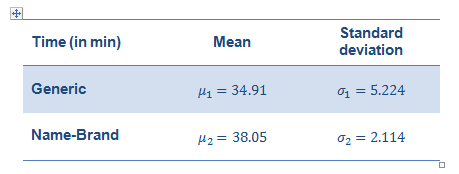We state the hypotheses as: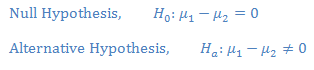Following assumptions are made:

The two groups are independent to each other.

The normal distribution (approx.) of population.

Given that the two groups have equal variances. Hence, we will use Pooled Standard Error Method. A Two-tailed, non-directional hypothesis test is used.

We will be using a t statistic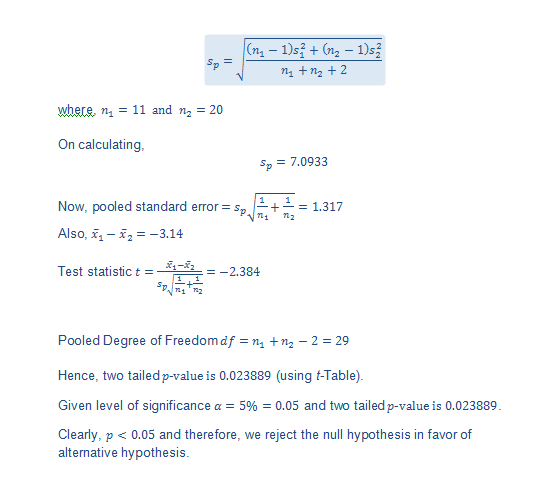Hence, we conclude that the generic drug does not have the same mean time until peak bioavailability as the name-brand drug.

b. Multiplier for a 95% confidence level is 1.96

Confidence interval for the mean difference is given by: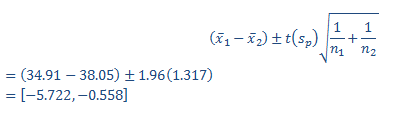c. We state the hypotheses as: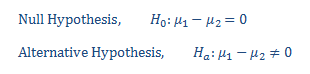Given that the groups have unequal variances: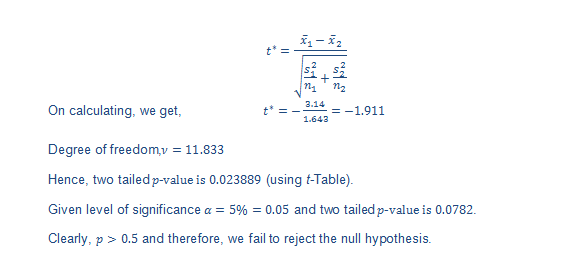Confidence interval for the mean difference is given by: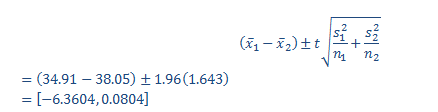Since, the confidence interval includes 0 we can say that there is no significant difference between the means of the two populations, at the given level of confidence.

d. The results of part (d) should be used as they are more valid. Since the standard deviation of two groups are not similar (one is even more than twice of the other), it is not a favorable situation to run the tests on assuming equal variances, as in part (b).

### Question 2

a. We calculate the standard deviation and mean of both sample populations;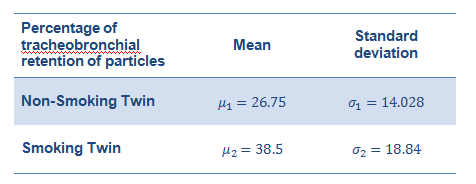b. For testing if there is a difference in the means of the two data sets, it is appropriate to use a two-tailed non-directional hypothesis test assuming equal variances, i.e.using Pooled Standard Error Method as the standard deviation of two groups is almost similar (one is not more than twice of the other).

c. We state the hypotheses as: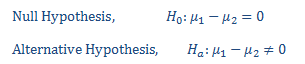Following assumptions are made:

• The two groups are independent to each other.
• The normal distribution (approx.) of population.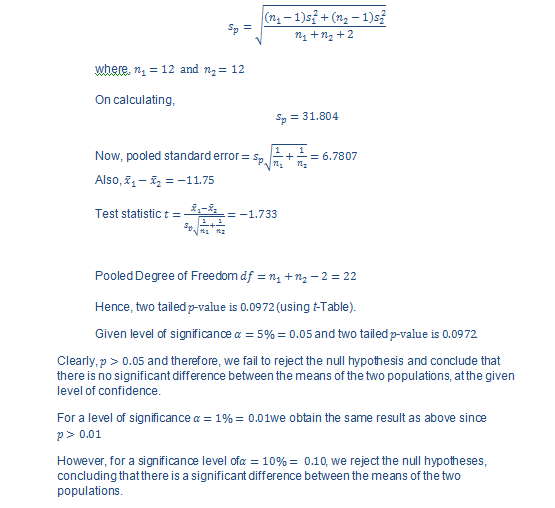d. Since the ‘Sig.’ value of the Shapiro-Wilk Test is greater than 0.05, the data sets are normally distributed. If it is below 0.05, the data significantly deviate from a normal distribution.The result of SPSS output is in support to the validity of assumption made in part (b).

### Question 3

a. We calculate the standard deviation and mean of all three sample populations;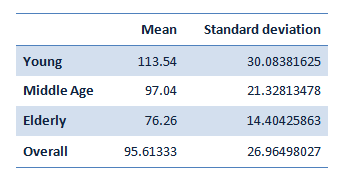b.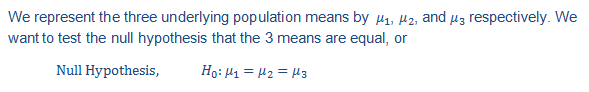The alternative hypothesis is that at least one of the population means differs from one of the others.
Assuming the normal distribution and the equal variances of all the three groups, we will conclude the results using one-way analysis of variance t-test.

Also n_1=n_2=n_3=6,k = 3 and n = 18 (the sample sizes for the three groups are same).

The overall sample mean is: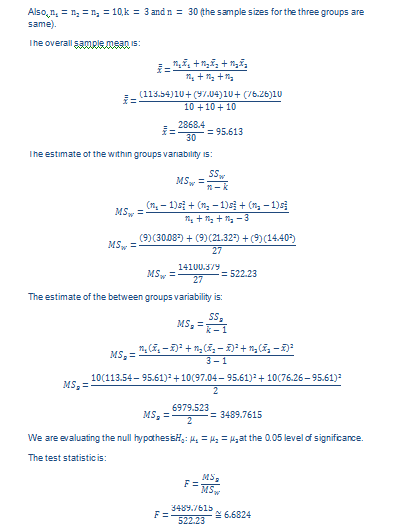The estimate of the within groups variability is: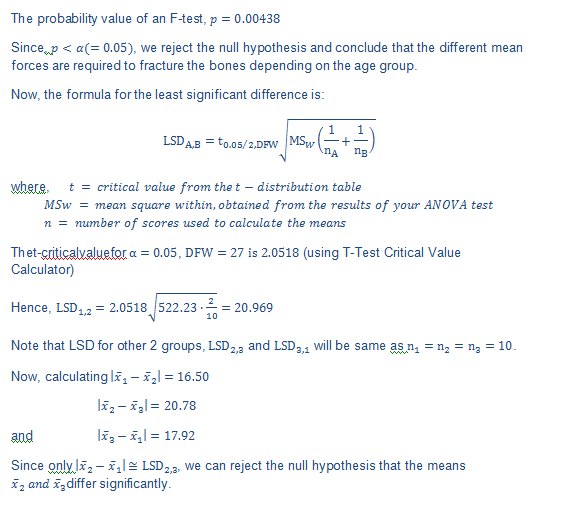The estimate of the between groups variability is: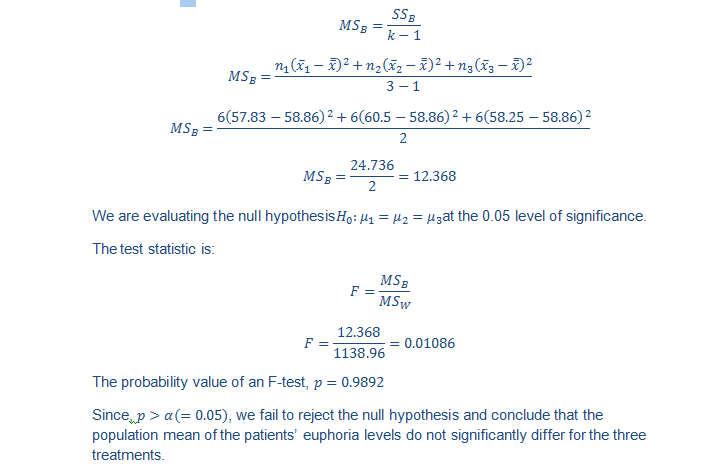c. The ‘Tests of Normality’of the SPSS output is appropriate as the ‘Sig.’ value of the Shapiro-Wilk Test is greater than 0.05, which is in accordance to our assumption that the data sets are normally distributed. If it is below 0.05, the data significantly deviate from a normal distribution.

Also, the ‘Test of Homogeneity of Variances’ of the SPSS output is appropriate in accordance with the assumption made that all groups have equal variances.

### Question 4

a. We calculate the standard deviation and mean of all three sample populations;b.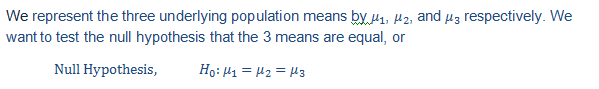The alternative hypothesis is that at least one of the population means differs from one of the others.
Assuming the normal distribution and the equal variances of all the three groups, we will conclude the results using one-way analysis of variance t-test.The test statistic is:c. The ‘Tests of Normality’ can be performed using the SPSS to check if the ‘Sig.’ value of the Shapiro-Wilk Test is greater than 0.05, which must be greater if in accordance to our assumption that the data sets are normally distributed. If it is below 0.05, the data significantly deviate from a normal distribution.
Also, the ‘Test of Homogeneity of Variances’ can be performed using the SPSS to support the underlying assumption that all groups have equal variances.